Function Repository Resource:

Symmetric tridiagonal matrix for Gaussian quadrature

Contributed by: Paul Abbott (additional contributions by Wolfram Research staff)
 ResourceFunction["GaussianQuadratureMatrix"][n] returns the n×n symmetric tridiagonal matrix that determines the abscissas and weights of the Gaussian quadrature.

## Details and Options

The abscissas and weights for the elementary n-point Gaussian quadrature formula are simply related to the eigenvalues and first component of the orthonormal eigenvectors of this matrix.

## Examples

### Basic Examples (1)

Compute the symmetric tridiagonal matrix of size 6×6:

 In:=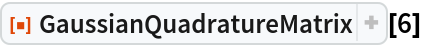Out=In:=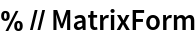Out=### Properties and Relations (3)

The abscissas and weights for the elementary n-point Gaussian quadrature are related to the eigensystem of the nn symmetric tridiagonal Gaussian quadrature matrix:

 In:=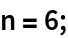In:=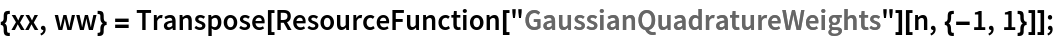In:=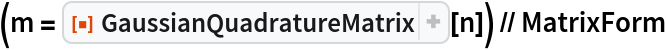Out=Eigenvalues of the matrix determine the abscissas:

 In:=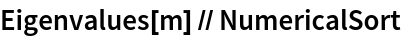Out=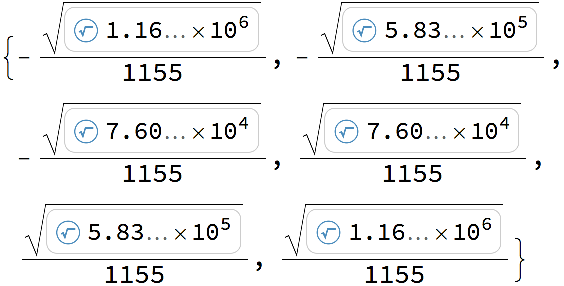In:=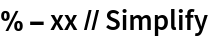Out=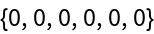Eigenvectors of the matrix determine the weights:

 In:=In:=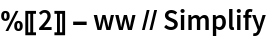Out=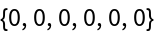## Version History

• 1.0.0 – 06 November 2020